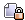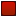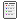###Author Topic: Population Model  (Read 3018 times)

####Hooman

• Hero Member
••• Posts: 4951##### Population Model
« on: October 28, 2005, 07:53:12 PM »
I'm reposting the following since it was lost when the forums went down. (Luckily I had this one saved to a text file.)

So, wouldn't you like to know how Outpost2 calculation population growth and population... decay? Well, I thought I'd include how it's done here.

It's interesting to note that at no point does your population rely (directly) on random numbers. So in that sense it is fair. If you take care of your colony at least as well as someone else, you should have at least as many colonists as them. The only possibility for "randomness" is how morale affects your population, but morale is also not exactly very random, and is certainly pretty close to fair. (More on that at a later time). For the purpose of this discussion, I will ignore casualties due to building loses, and deal only with the natural changes in population levels.

The following discussion makes use of M_FERT_RATE and M_DIE_RATE from morale.txt. M_FERT_RATE determines the rate at which new kids are born. A higher value means a higher birth rate. M_DIE_RATE determines the rate at which people (kids, workers, and scientists) die. A HIGHER value means a LOWER death rate.

The population of you colony (kids, workers, and scientists) are updated in the following manner (and order).

New workers are trained from kids.
If the number of kids you have is greater than 0, and if you have an active university then kids will be trained into workers. (Having more than 1 university has no affect here). First, add your current number of kids to "workerGrowthRemainder". This value keeps track of the fractional number of workers that you have trained so far. This is what allows for worker growth across multiple growth periods, even though at no single interval do you get enough to produce one new "entire" worker. Now, determine the "totalPopulation" (numKids + numWorkers + numScientists). Cap the total population at 160. So "cappedPopulation" = CAPPED

(160, totalPopulation). This value is actually used to limit your population's rate of growth as it gets larger, but will only limit it up to a certain point. Now consider the fraction: workerGrowthRemainder / (((cappedPopulation/40)*3 + 36) * 4). The quotient (interger portion) of this value is the number of new workers trained. The remainder is your new workerGrowthRemainder. The value of workerGrowthRemainder is stored for reuse the next time the population is updated.

Now, workerGrowthRemainder (as are all remainders soon to be discussed) are initially set to 0 when the game starts. If say you had 30 kids, and the denominator on the above fraction was 100 (for the sake of demonstration here), then after 3 periods of population growth, your workerGrowthRemainder would have grown to 90, but you still would not have had a new worker trained. During the next population growth cycle, the workerGrowthRemainder would have increased to 120, which now gives a result of 1 new worker trained, and a new starting value for workerGrowthRemainder of 20. Growth continues from there by adding your (new) current number of kids that the workerGrowthRemainder each cycle.

Now, when your number of workers is increased, it will of course decrease your number of kids by the corresponding amount.

Next up, it calculates the number of new kids.
If numWorkers + numScientists > numKids and you have an active nursery, then new kids will be born (or fractionally born  :lol: ). (Having more than 1 nursery has no effect here). First, kidGrowthRemainder is increased by (numWorkers/2 + numScientists/4). Then consider the fraction kidGrowthRemainder / M_FERT_RATE. The quotient is the number of new kids born, and the remainder is your new starting kidGrowthRemainder for the next cycle.

New scientists can only be obtained from training them at a university, so there is no scientists growth to be discussed here.

Next up is kid deaths.
If your number of kids is greater than 0, you will have kids that die (or are fractionally dead :lol: ). First, kidDeathRemainder is increased by numKids. Then, the effectiveness of nurseries at preventing kid deaths is calculated. This is numActiveNurseries * 40. Also, M_DIE_RATE/2 is taken into consideration. Now, consider the fraction: kidDeathRemainder / (totalMedCenterCapacity + numActiveNurseries * 40 + M_DIE_RATE/2). The quotient is the number of kids that die, and the remainder is the new kidDeathRemainder for the next cycle.

Note: Having multiple nurseries has a beneficial effect here. More nurseries means the denominator of the fraction will be bigger, thus lowering the number of deaths. However, a unupgraded med center has a capacity of 40 (which is essentially the capacity of the nursery in this context) and takes just as many workers, and scientst, as well as just as much power. So you'd be better off having multiple med centers rather than multiple nurseries, since med center will help reduce the death rate for workers and scientists too. Plus, once you upgrade med centers, they have a capacity of 75, and so they are much more effective than an nursery.

Note: Since M_DIE_RATE/2 is used here instead of M_DIE_RATE, kids will have a death rate of (essentially) twice that of an adult (worker or scientist). Existence of nurseries or med centers will skew this a little.

Next up is worker deaths.
If numWorkers > 0 then some workers will die (or fractionally die). First, workerDeathRemainder is increased by numWorkers. Then, consider the fraction: workerDeathRemainder / (totalMedCenterCapacity + M_DIE_RATE). The quotient is the number of dead workers, and the remainder is the new workerDeathRemainder for the next cycle.

And finally, we have scientist deaths. (Analogous to worker deaths.)
If numScientists > 0 then some scientists will die (or fractionally die). First, scientistDeathRemainder is increased by numScientists. Then, consider the
fraction: scientistsDeathRemainder / (totalMedCenterCapacity + M_DIE_RATE). The quotient is the number of dead workers, and the remainder is the new scientistsDeathRemainder for the next cycle.

So there you have it. The population growth/decay model as used by OP2.